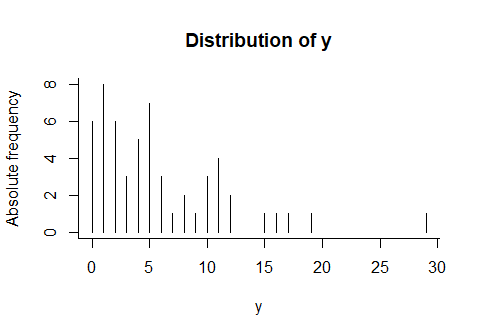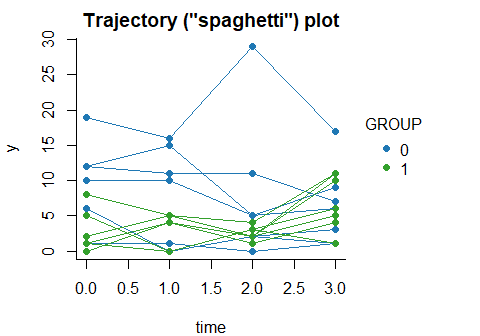# Introduction

## What’s ptmixed?

ptmixed is an R package that has been created to estimate the Poisson-Tweedie mixed effects model proposed by Signorelli, Spitali and Tsonaka (2020, in press). Poisson-Tweedie mixed-effects model: a flexible approach for the analysis of longitudinal RNA-seq data. To appear in Statistical Modelling (arXiv preprint: arXiv:2004.11193). The Poisson-Tweedie mixed effects model is a generalized linear mixed model (GLMM) for count data that encompasses the negative binomial and Poisson GLMMs as special cases. It is particularly suitable for the analysis of overdispersed count data, because it allows to model overdispersion, zero-inflation and heavy-tails more flexibly than the negative binomial GLMM.

The package comprises not only functions for the estimation of the Poisson-Tweedie mixed model, but also functions for the estimation of the negative binomial and Poisson-Tweedie GLMs and of the negative binomial GLMM, alongside with some (simple) data visualization functions.

## Get started

The package can be installed directly from CRAN using

install.packages('ptmixed')

After installing the package, you can load its functionalities through

library('ptmixed')

# An overview of the package functionalities

The package comprises different types of functions:

• functions for the estimation of the Poisson-Tweedie and negative binomial GLMMs: see ?ptmixed and ?nbmixed
• functions for the estimation of the Poisson-Tweedie and negative binomial GLMs: see ?ptglm and ?nbglm
• functions to summarize model fits: see ?summary.ptglmm and ?summary.ptglm
• a function that allows to carry out univariate and multivariate Wald tests: see ?wald.test
• a function to compute the predicted random effects for a fitted Poisson-Tweedie or negative binomial GLMM: see ?ranef
• functions for data visualization: see ?pmf and ?make.spaghetti

# A step by step example

In this section I am going to present a step by step example whose aim is to show how the R package ptmixed can be used to estimate the Poisson-Tweedie GLMM, as well as a few simpler models.

## Data preparation

All functions in the package assume that data are in the so-called ‘’long format’’. Let’s generate an example dataset (already in long format) using the function simulate_ptglmm:

example.df = simulate_ptglmm(n = 14, t = 4, seed = 1234,
beta = c(2.3, -0.9, -0.2, 0.5),
D = 1.5, a = -1,
sigma2 = 0.7)
## Loading required namespace: tweeDEseq
data.long = example.df$data head(data.long) ## y id group time ## 1 6 1 0 0 ## 2 0 1 0 1 ## 3 2 1 0 2 ## 4 1 1 0 3 ## 5 10 2 0 0 ## 6 10 2 0 1 In this example I have generated a dataset of 14 subjects with 4 repeated measurements each. y is the response variable, id denotes the subject identicator, group is a dummy variable and time is the time at which a measurement was taken. ## Data visualization Before fitting a model, it is often useful to make a few plots to get a feeling of the data that you would like to model. Below I use two simple plots to visualize the distribution of the response variable and its relationship with the available covariates. We can view the marginal distribution of the response variable y using the function pmf, and visualize the individual trajectories of subjects over time using the function make.spaghetti: pmf(data.long$y, xlab = 'y', title = 'Distribution of y')make.spaghetti(x = time, y = y, id = id,
group = group, data = data.long,
title = 'Trajectory ("spaghetti") plot',
legend.title = 'GROUP')## The Poisson-Tweedie generalized linear mixed model

The most important function of the package, ptmixed, is a function that makes it possible to carry out maximum likelihood (ML) estimation of the Poisson-Tweedie GLMM. This function employs the adaptive Gauss-Hermite quadrature (AGHQ) method to evaluate the marginal likelihood of the GLMM, and then maximizes this likelihood using the Nelder-Mead and BFGS methods. Finally, if hessian = T (default value) a numerical evaluation of the hessian matrix (needed to compute the standard errors associated to the parameter estimates) in correspondance of the ML estimate is performed.

### Model Estimation

Estimation of the Poisson-Tweedie GLMM can be carried out using ptmixed:

pt_glmm = ptmixed(fixef.formula = y ~ group*time, id = id,
data = data.long, npoints = 3,
hessian = T, trace = F)
## Loading required namespace: GLMMadaptive
## Loading required namespace: moments
## Loading required namespace: lme4
## Loading required namespace: mvtnorm
##
##
## Total number of NM iterations = 570
## Convergence reached. Computing hessian...
## Loading required namespace: numDeriv

The code above requires to estimate a GLMM

• with y as response, group, time and their interaction as fixed effects (as specified in fixef.formula), and a subject-specific random intercept (id = id)
• using an AGHQ with 3 quadrature points (npoints = 3)
• and to further evaluate the Hessian matrix at the MLE (hessian = T)

Note that the function comprises several other arguments, detailed in the function’s help page. In particular, there are four remarks that I’d like to make here:

• an offset term, if relevant, can be included through the offset argument
• a higher number of quadrature points can be specified by changing the value of npoints (my recommendation is to use npoints = 5)
• detailed information about the optimization can be printed on screen by specifying trace = T (here I have set trace = F to prevent a long tracing output to be printed in the middle of the vignette)
• ML estimation for this model is not trivial, and optimizations may sometimes fail to converge. If this happens, you may try to use a different number of quadrature points (npoints), to change the default values of the arguments freq.updates, reltol, maxit and min.var.init, or to supply an alternative (sensibly chosen) starting value (theta.start)

### Viewing parameter estimates, standard errors and p-values

The results of the fitted model can be viewed using

summary(pt_glmm)
## Loading required namespace: matrixcalc
## Loglikelihood: -140.623
## Parameter estimates:
##             Estimate Std. error       z p.value
## (Intercept)   2.0059     0.2865  7.0010  0.0000
## group        -1.4523     0.4641 -3.1292  0.0018
## time         -0.1359     0.0762 -1.7826  0.0746
## group:time    0.5105     0.1458  3.5019  0.0005
##
## Dispersion = 1.63
## Power = -0.23
## Variance = 0.41

that reports the ML estimates of the regression coefficients (column Estimate), the associated standard errors (column Std. error) and univariate Wald tests (columns z and p.value), as well as the ML estimates of the dispersion and power parameters of the Poisson-Tweedie distribution, and the ML estimate of the variance of the random effects.

### Testing more complex hypotheses

More complex hypotheses can be tested using the multivariate Wald test (or, when possible, the likelihood ratio test).

For example, one may want to test the null hypothesis that there are no differences between the two groups, that is to say that the regression coefficients of group and group:time are both = 0.

To test this hypothesis we first need to specify it in the form $$L \beta = 0$$, where $$L$$ is specified as follows:

L.group = matrix(0, nrow = 2, ncol = 4)
L.group[1, 2] = L.group[2, 4] = 1
L.group
##      [,1] [,2] [,3] [,4]
## [1,]    0    1    0    0
## [2,]    0    0    0    1

Then, we can proceed with computing the multivariate Wald test:

wald.test(pt_glmm, L = L.group, k = c(0, 0))
## Loading required namespace: aod
##       chi2 df            P
## 1 14.22634  2 0.0008143085

### Computing the predicted random effects

To computate the predicted random effects, simply use

ranef(pt_glmm)
##           1           2           3           4           5           6
## -0.74838728  0.31472249  1.13391844 -1.17000951  0.49289844  0.40439346
##           7           8           9          10          11          12
## -0.22676683  0.31639414 -0.35419327 -0.02760355  0.17689645 -0.27431201
##          13          14
## -0.18584234  0.65265844

## Simpler models

The Poisson-Tweedie GLMM is an extension of three simpler models:

• negative binomial GLMM (this is obtained by fixing the power parameter a = 0)
• Poisson-Tweedie GLM (this is obtained by removing the random effects from the model)
• negative binomial GLM (this is obtained by fixing the power parameter a = 0 and removing the random effects from the model)

For this reason, the package also offers the possibility to estimate these simpler models, as illustrated below.

### Negative binomial generalized linear mixed model

The syntax to estimate the negative binomial GLMM is the same as that used for the Poisson-Tweedie GLMM. Just make sure to replace the function ptmixed with nbmixed:

nb_glmm = nbmixed(fixef.formula = y ~ group*time, id = id,
data = data.long, npoints = 3,
hessian = T, trace = F)
##
## Total number of iterations = 362
## Convergence reached. Computing hessian...

To view the model summary and compute the predicted random effects, once again you can use

summary(nb_glmm)
## Loglikelihood: -140.623
## Parameter estimates:
##             Estimate Std. error       z p.value
## (Intercept)   1.9855     0.2890  6.8699  0.0000
## group        -1.4244     0.4645 -3.0666  0.0022
## time         -0.1338     0.0761 -1.7579  0.0788
## group:time    0.5057     0.1442  3.5067  0.0005
##
## Dispersion = 1.63
## Power = 0
## Variance = 0.41
ranef(nb_glmm)
##           1           2           3           4           5           6
## -0.73856990  0.33249113  1.15104776 -1.16599968  0.51121344  0.42102715
##           7           8           9          10          11          12
## -0.21148564  0.31620528 -0.35946848 -0.02816143  0.17458088 -0.27611762
##          13          14
## -0.18756259  0.65244576

### Poisson-Tweedie generalized linear model

Estimation of the Poisson-Tweedie GLM can be done using the ptglm function:

pt_glm = ptglm(formula = y ~ group*time, data = data.long, trace = F)
summary(pt_glm)
## Loglikelihood: -152.931
## Parameter estimates:
##             Estimate Std. error       z p.value
## (Intercept)   2.2396     0.2128 10.5265  0.0000
## group        -1.3792     0.4083 -3.3778  0.0007
## time         -0.1661     0.1244 -1.3353  0.1818
## group:time    0.5111     0.2046  2.4984  0.0125
##
## Dispersion = 4.24
## Power = -0.16

### Negative binomial generalized linear model

Finally, estimation of the negative binomial GLM can be done using the nbglm function:

nb_glm = nbglm(formula = y ~ group*time, data = data.long, trace = F)
summary(nb_glm)
## Loglikelihood: -152.955
## Parameter estimates:
##             Estimate Std. error       z p.value
## (Intercept)   2.2401     0.2146 10.4362  0.0000
## group        -1.3751     0.4070 -3.3788  0.0007
## time         -0.1701     0.1241 -1.3701  0.1707
## group:time    0.5170     0.2030  2.5473  0.0109
##
## Dispersion = 4.37
## Power = 0

# Further details and material

The aim of this vignette is to provide a quick-start introduction to the R package ptmixed. Here I have focused my attention on the fundamental aspects that one needs to use the package.

Further details, functions and examples can be found in the manual of the package.

The description of the method is available in an article that you can read here.